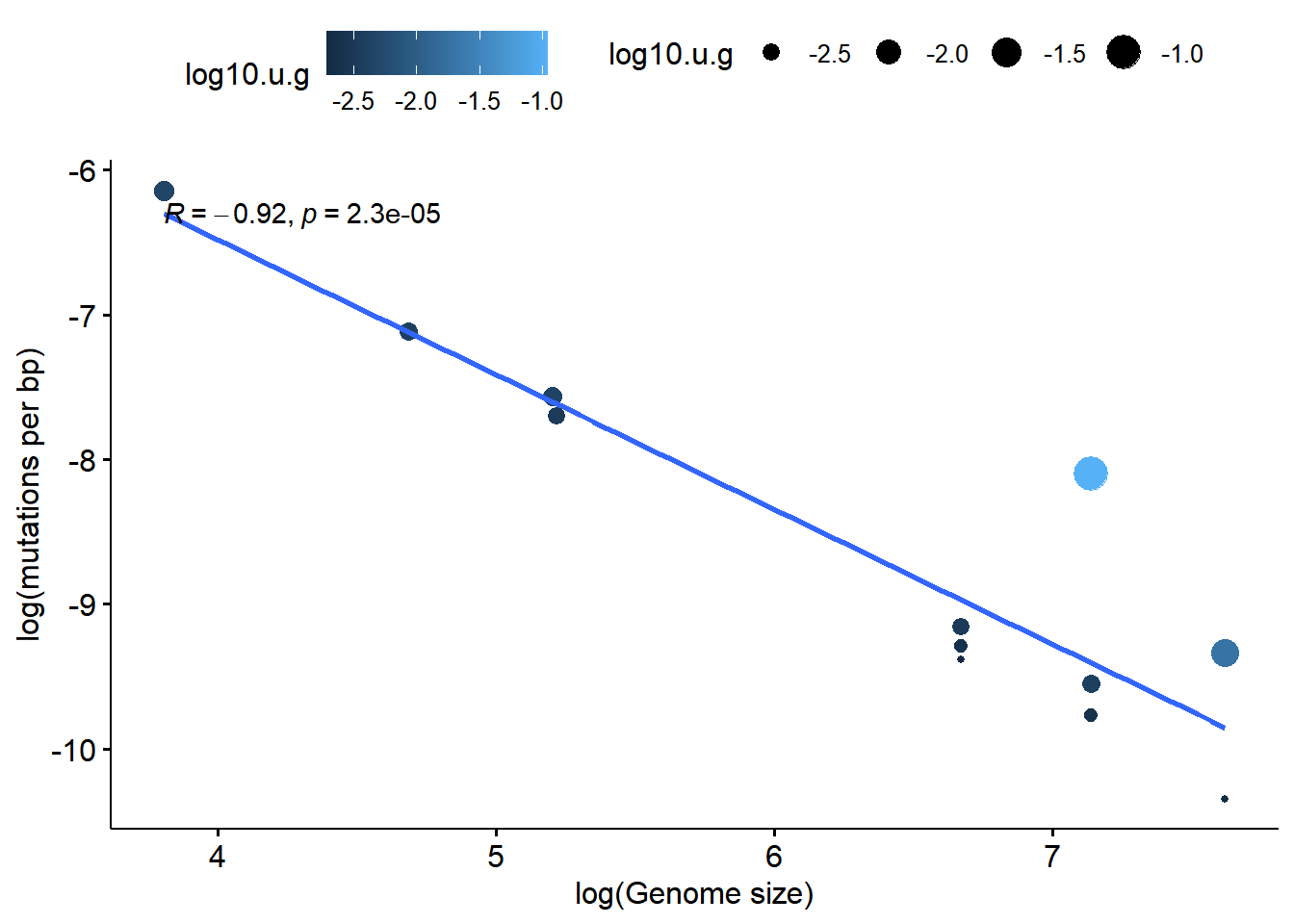## Introduction

This exercise is meant to challenge you to build a dataframe by hand in R. It is based off of the Table 1 in Drake (1991) “A constant rate of spontaneous mutation in DNA-base microbes” (PNAS August 15, 1991 88 (16) 7160-7164; https://doi.org/10.1073/pnas.88.16.7160)

There are two parts to this tutorial. This versions will guide you through the process of building the dataframe and exploring it. A second version is designed to challenge your understanding of the steps we went through.

## Functions, concepts and vocab

### Functions

c() rep() is is.vector is.matrix class length nchar == round() data.frame() log() log10() ggpubr::ggscatter()

### Concepts:

Scientific notation in R Encoding information on a plot with size and color

### Vocab

smoother correlation

ggpubr

## Preliminaries

### Non-R materials

For this you’ll need a copy of Drake (1991), which can be found at https://www.pnas.org/content/88/16/7160

We will focus on rebuiling Table 1 and Figure 1 entirely in R without importing the data from a spreadsheet.

### Packages

The only package you will need is ggpubr. Load ggpubr using library():

library(ggpubr )

## The data

Table 1 of Drake (1991) has the following columns. Note that the symbol mu (u) is frequently used to represent mutation rates.

1. A list of organisms (viruses, bacteria and invertebrate eukaryotes)
2. genome sizes (G) for each organism, in scientific notation
3. A target gene, which was assessed for the occurrence of mutations
4. An estimate of the mutation rate per bases (bp) of the genome. This gets log transformed and used as the y variable in Figure 1. I’ll use the symbol u.bp for this variable
5. An estimate of the mutation rate per genome (u.g). This variable isn’t in scientific notation so we’ll work with it first because its a bit easier to read.

The organism column has gaps in it because there are multiple target genes for these species

1. 3 E. coli genes
2. 3 S. cerevisiae genes
3. 2 N. crassa genes

Note that the very last numbers that appear in the mutation columns are means, not data!

## Creating vectors in R

We can build up a dataframe in R by making a vector for each column. This is a bit tedious but will build up our skills, so be patient.

We can make a vector of organism names like this. We need to make sure each word representing an organisms is in quotes, and that there is a comma after each word.

org <- c("M13","lamb", "T2", "T4", "EC","EC","EC","SC","SC","SC","NC","NC")

We can use line breaks while defining an R object, which can make it easier to keep track of what were typing. The following code is equivalent to what we just ran. Because there are multiple E. coli (EC) , SC and NC, I’m putting them on their own lines.

org <- c("M13", "lamb", "T2", "T4",
"EC", "EC", "EC",
"SC", "SC", "SC",
"NC","NC")

If you want to be fancy, use rep() for the repeated elements.

org <- c("M13","lamb", "T2", "T4",
rep("EC",3),
rep("SC",3),
rep("NC",2))

As always we need to check to make sure that the object we’re making is what we think it is and represents what we want. Use the following commands to check on the object:

• is
• is.vector
• is.matrix
• class
• length
• nchar
is(org)
##  "character"           "vector"              "data.frameRowLabels"
##  "SuperClassMethod"

Next let’s make the next vector. Below is the code you’ll need, but I’ve left off the last 3 numbers. Paste the code into the code chunk and add the necessary numbers from the original table in Drake (1991). Don’t forget the commas! Also note that numbers don’t get quotes around them.

G.p <- c(6.41, 4.85, 1.60, 1.66, 4.70, 4.70, 4.70, 1.38, 1.38 )

# Write the code to make the G.p vector here
# be sure to add the necessary numbers

G.p <- c(6.41, 4.85,1.60, 1.66,
4.70, 4.70, 4.70,
1.38, 1.38,1.38,
4.19,4.19)

Again, we explore this. Run these functions and note the output

• is
• class
• length
• nchar
is(G.p)
##  "numeric" "vector"

## Checking the length of vectors

Vectors in R have length, not dimension, so to see how big they are we use the length() command; dim() won’t work. Neither will ncol() or nrow().

All of our columns have the same number of elements in them. We can make sure we don’t have any errors in our data entry by using a logical operation, ==, to confirm that the length of each vector is the same. This is very useful when vectors are big and/or when we’re writing code to automate a process

First, check the length of one of the vectors

length(G.p)
##  12
length(org)
##  12

Next, run this code to check that the two lengths are the same: length(G.p) == length(org)

length(G.p)==length(org)
##  TRUE

## Exponents and scientific notation

To take the exponent of something in R use the up caret ^

So, if I want to type 1 million I can do this

1000000
##  1e+06

or I can do this

1*10^6
##  1e+06

Note that the output is in “e” notation. I could type this if I wanted

1e+06
##  1e+06

In the chunk below, write a logical expression using == to confirm that 1000000 is equal to 1*10^6. Write another one to check that 1e+06 is the same as 1000000.

1000000==1*10^6
##  TRUE
1000000==1e+06
##  TRUE

To represent the genome size column I could do this (just showing first 3 numbers)

G.with.10 <- c(6.41*10^5, 4.85*10^4, 1.60*10^5)

I could also do this

G.with.e <- c(6.41e+05, 4.85e+04, 1.60e+05)

I can confirm that that they are the same like this

G.with.10 == G.with.e
##  TRUE TRUE TRUE

When typing up this data I found it easier to break up the columns containing exponents into multiple columns, otherwise I was getting cross eyed.

One way to do this would be to split each number into what I’m calling a “prefix” and a “suffix”. Above we made a vector called G.p, which is the prefix of these numbers - the part before the multiplication symbol.

We can make another vector that contains the suffix - the 10^x part (or the 1e+0x part).

G.s <- c(10^3, 10^4,    10^5, 10^5, 10^6, 10^6, 10^6, 10^7, 10^7, 10^7, 10^7, 10^7)

We can then multiple these together and get the value we want

G.p*G.s
##       6410    48500   160000   166000  4700000  4700000  4700000 13800000
##   13800000 13800000 41900000 41900000

Note that this is doing is multiplying each element of G.p by the corresponding element of G.s. We could break this up like this

G.p*G.s
##  6410
G.p*G.s
##  48500

This works pretty well, but I found typing all those exponents to still be a little annoying, especially since many get repeated. I think its easier to just type the exponent. We’ll call this object “G.exp” for exponent

G.exp <- c(3, 4,    5, 5, 6, 6, 6, 7, 7, 7, 7, 7)

Again, if you want to be fancy you can play around with the rep() function, though this is optional

G.exp <- c(3, 4,
rep(5, 2),
rep(6, 3),
rep(7, 5))

Now, to get the numbers we want, we can do this: G.p*10^G.exp Can you figure out what’s going on? If not, check the next chunk

G.p*10^G.exp
##       6410    48500   160000   166000  4700000  4700000  4700000 13800000
##   13800000 13800000 41900000 41900000

Here I’ve added some parentheses to clarify the order of operations

 G.p*(10^G.exp)
##       6410    48500   160000   166000  4700000  4700000  4700000 13800000
##   13800000 13800000 41900000 41900000

If its still not clear, then maybe this will help:

G.s == 10^G.exp
##   TRUE TRUE TRUE TRUE TRUE TRUE TRUE TRUE TRUE TRUE TRUE TRUE

Let’s make a final G vector with genome sizes. This is the “prefix” I made (G.p) multipled by 10 raised to the exponent I defined in the vector G.exp.

G <-  G.p*(10^G.exp)

We can make a basic plot showing the distribution of genome sizes using the histogram function in R, hist().

hist(G)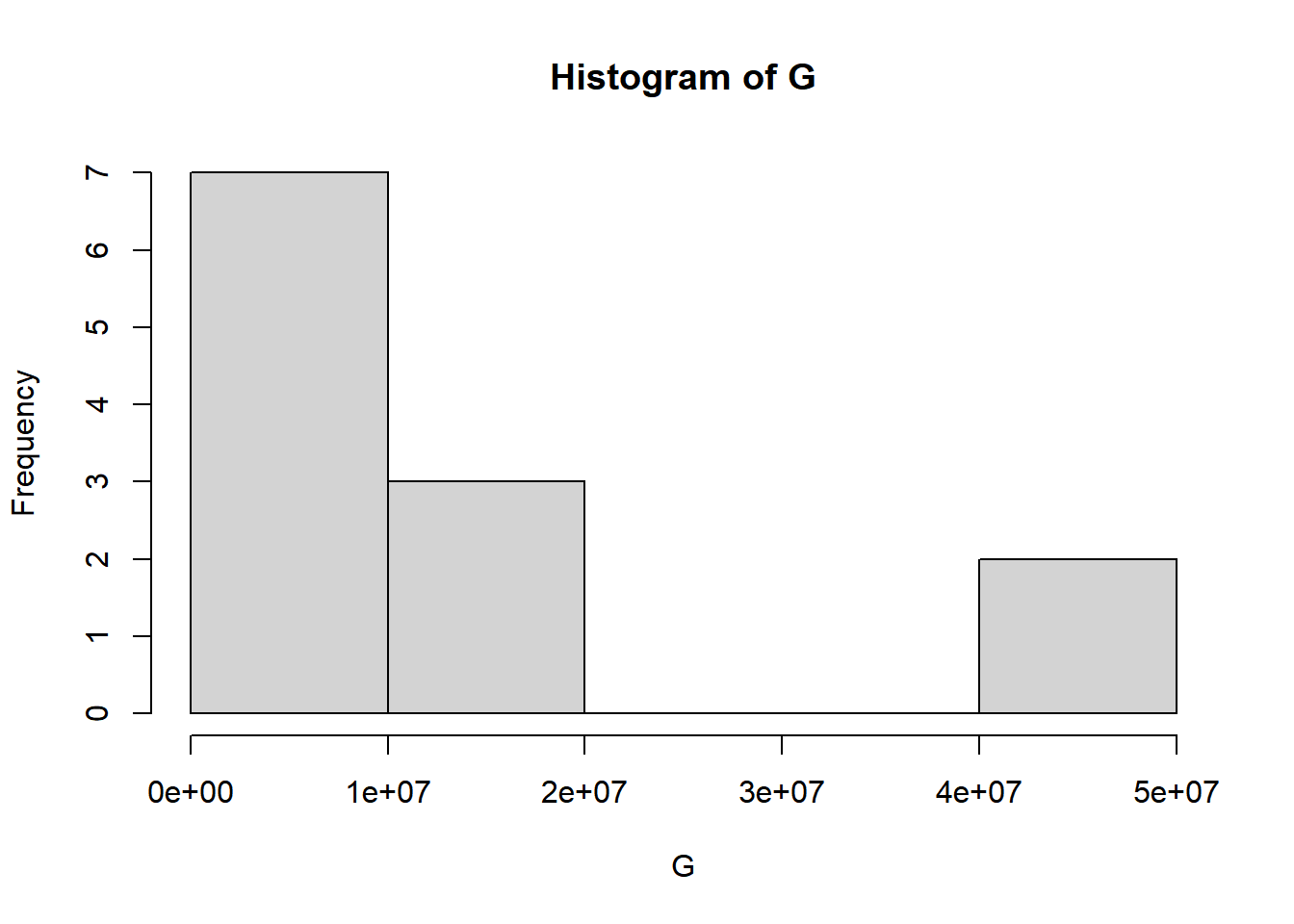The far left value is 0e+00, which just means 0.0. The first tick mark is 1e+07. Write this out using an exponent symbol ^ below:

1*10^7
##  1e+07

How do you interpret this graph? If you aren’t comfortable interpretting histograms see datavizcatalogue.com/methods/histogram.html and https://en.wikipedia.org/wiki/Histogram

Another way to look at this data would be with a boxplot

boxplot(G)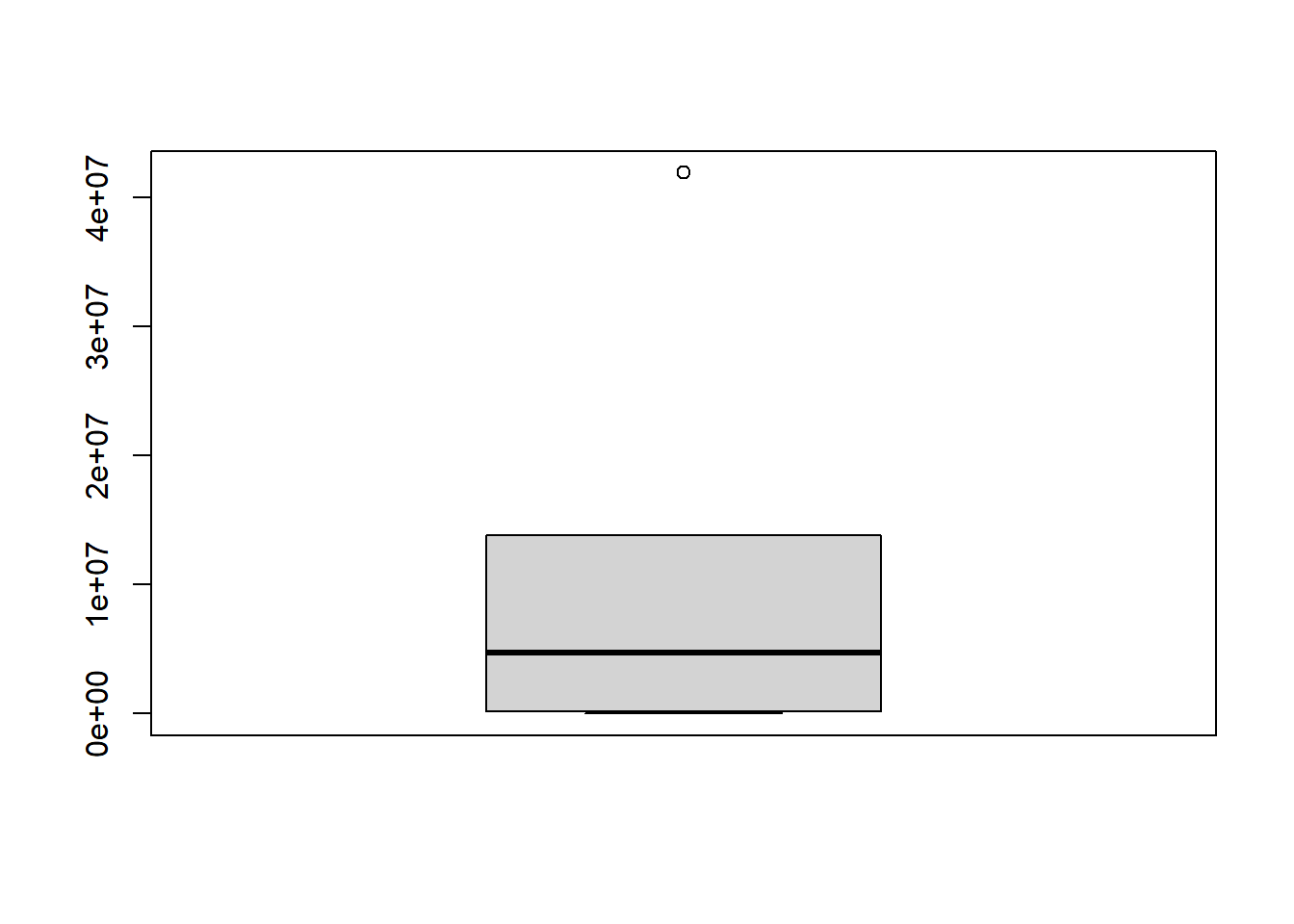This is actually a realy ugly plot, but whatever.

hist() and boxplot() are great for quick and dirty plots. Normally though I’ll use ggpubr to make plots using gghistogram() and ggboxplot().

## Some more vectors

Next we have the per genome mutation rate. The “u” is a stand-in for “mu” which is commonly used for mutation rates. So “u.bp” reads “mutation rate in bp.” First let’s do the “prefix” and assign it to a vector u.bp.p (mutation rate in bp prefix).

u.bp.p <- c(7.2, 7.7, 2.7, 2, 4.1, 6.9, 5.1, 2.8, 7.9, 1.7, 4.5, 4.6)

Confirm that this vector is the exact same size as our previous ones (Hint: requires “=”)

length(u.bp.p)==length(org)
##  TRUE

Confirm that what you just made was a vector. (Hint: what “is” it?)

is(u.bp.p)
##  "numeric" "vector"

Now let’s do the exponent and save it to a vector u.bp.exp.

u.bp.exp <- c(-7,
-8, -8,-8,
-10,-10,-10,-10,
-9,
-10,
-11,
-10)

For those who want to be fancy, can you do this using the rep() command? (Totally optionall).

As before, make sure this new vector u.bp.exp is the right length

length(u.bp.exp)
##  12

Now, ,ake the final column by carrying out the math. To do this put the following components together corrctly

• The “prefix” u.bp.p
• The caret ^
• The exponent u.bp.exp

Assign the result to an object u.bp. Try to type this out yourself, or cut and paste it if needed:

u.bp <- u.bp.p*10^u.bp.exp

u.bp <-u.bp.p*10^u.bp.exp

As always, check that the size of u.bp is correct

length(u.bp)
##  12

The last column of Drake (1991) Table 1 is the per-genome mutation rate, u.g.

u.g <- c(0.0046, 0.0038, 0.0043,
0.0033, 0.0019, 0.0033,
0.0024, 0.0038, 0.11,
0.0024, 0.0019, 0.019)

We can confirm that the vector is that same size as the prevous ones

length(G.s) == length(u.g)
##  TRUE

I’m not 110% sure about the methods for this paper. I’m wondering if the per genome mutation rate u.g can be calculated from the per base pair mutation rate (u.bp) and the genome size (G). How do you think you could do this calculation in terms of the biology, the math, and in R? Save the output as an object u.g.2 (2nd version of u.g).

Hint: Don’t over think it; there’s just one mathematical operation, either multiplication or division (The answer is a bit further down).

u.g.2 <-G*u.bp

Some more hints: G is the size of each genome. So the first genome in our vector has the size in base pairs (bp).

G
##  6410

u.bp is the rate of mutations per base pairs. The mutation rate per base pairs for the first genome in the vector is

u.bp
##  7.2e-07

So, if we want to determine the number of mutations per genome, we multiply the size of the genome in bp by the per bp rate of mutation

G*u.bp
##  0.0046152

We can compare this to what is in the table as the per genome rate of mutation (u.g)

u.g
##  0.0046

We can do all the multiplication of the whole vector in one shot like this: u.g.2 <- G*u.bp

Try to type this first; if you have trouble, copy and paste this into the code chunk below.

 u.g.2 <- G*u.bp   

So we have the value of u.g from the column in Table 1 that we typed up above, and now we have our attempt to re-calculate it. One way to check them against each other is to make a simple dataframe and compare by eye:

data.frame(u.g, u.g.2)
##       u.g     u.g.2
## 1  0.0046 0.0046152
## 2  0.0038 0.0037345
## 3  0.0043 0.0043200
## 4  0.0033 0.0033200
## 5  0.0019 0.0019270
## 6  0.0033 0.0032430
## 7  0.0024 0.0023970
## 8  0.0038 0.0038640
## 9  0.1100 0.1090200
## 10 0.0024 0.0023460
## 11 0.0019 0.0018855
## 12 0.0190 0.0192740

This looks pretty close. Let’s round things off so its easier to read. Rounding is done with the round() command.

To round something in R, we need to give it a number (or numbers in a vector) and an indication of how many digits to round off to. In the original table the ubmres are rounded off to 4 digits

round(0.0037, 4)
##  0.0037

Instead of u.g.2, run the previous data.frame code with round(u.g.2, 4). That is, instead of u.g.2, put in round(u.g.2, 4)

data.frame(u.g,round(u.g.2,4))
##       u.g round.u.g.2..4.
## 1  0.0046          0.0046
## 2  0.0038          0.0037
## 3  0.0043          0.0043
## 4  0.0033          0.0033
## 5  0.0019          0.0019
## 6  0.0033          0.0032
## 7  0.0024          0.0024
## 8  0.0038          0.0039
## 9  0.1100          0.1090
## 10 0.0024          0.0023
## 11 0.0019          0.0019
## 12 0.0190          0.0193

Things don’t round off perfectly, perhaps because of other rounding they did during their workflow. But there’s something weird with the first number. Either I made a type or something else is up. Let me know what you think!

Another useful comparison here is ==. See if you can predict what will happen when you run:

u.g == round(u.g.2, 4)

u.g == round(u.g.2, 4)
##    TRUE FALSE  TRUE  TRUE  TRUE FALSE  TRUE FALSE FALSE FALSE  TRUE FALSE

When doing comparisons like this, why might it be really important to consider rounding?

A: to check whether they are the same.

## Make a dataframe

We can put all our pieces together into a dataframe like this with data.frame():

table1 <- data.frame(organism = org,
G = G,
target = NA,
u.bp = u.bp,
u.g = u.g)

For each column I am specifying a name and telling it the vector to turn into a column. For target I’m telling it just to fill it in with NA, which means there is no data

As always, we want to check what we just made. Run at least 2 commands exploring the size, share or content of the datarame

dim(table1)
##  12  5
head(table1)
##   organism       G target    u.bp    u.g
## 1      M13    6410     NA 7.2e-07 0.0046
## 2     lamb   48500     NA 7.7e-08 0.0038
## 3       T2  160000     NA 2.7e-08 0.0043
## 4       T4  166000     NA 2.0e-08 0.0033
## 5       EC 4700000     NA 4.1e-10 0.0019
## 6       EC 4700000     NA 6.9e-10 0.0033

## Make Figure 1 with base R

We can make figure 1 with the basic R plot() command. FIrst let’s plot the raw data.

plot(u.bp ~ G, data = table1)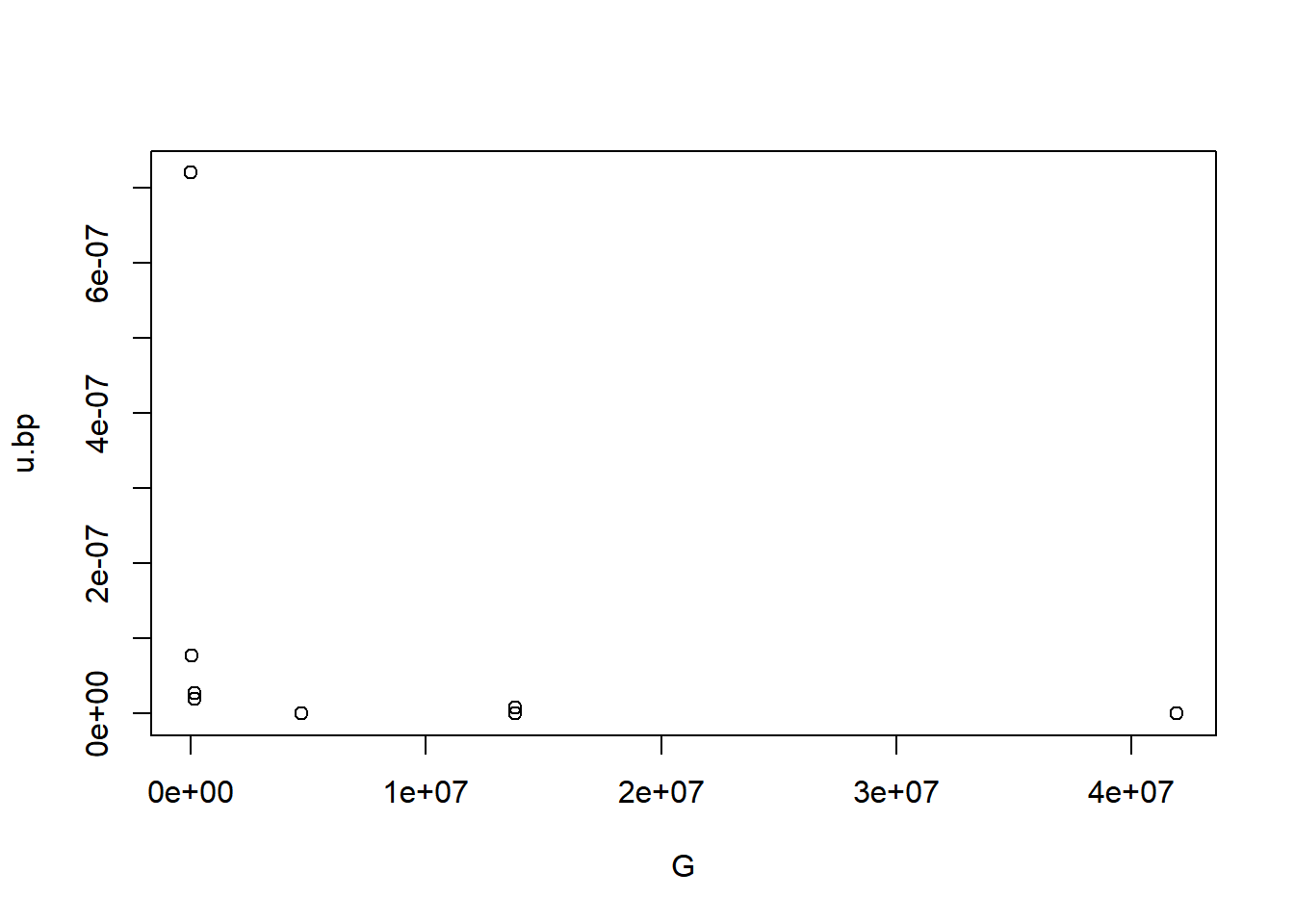If you look at the figure its actually on the log scale. We can nest the log function within the plot function like this

plot(log(u.bp) ~ log(G), data = table1)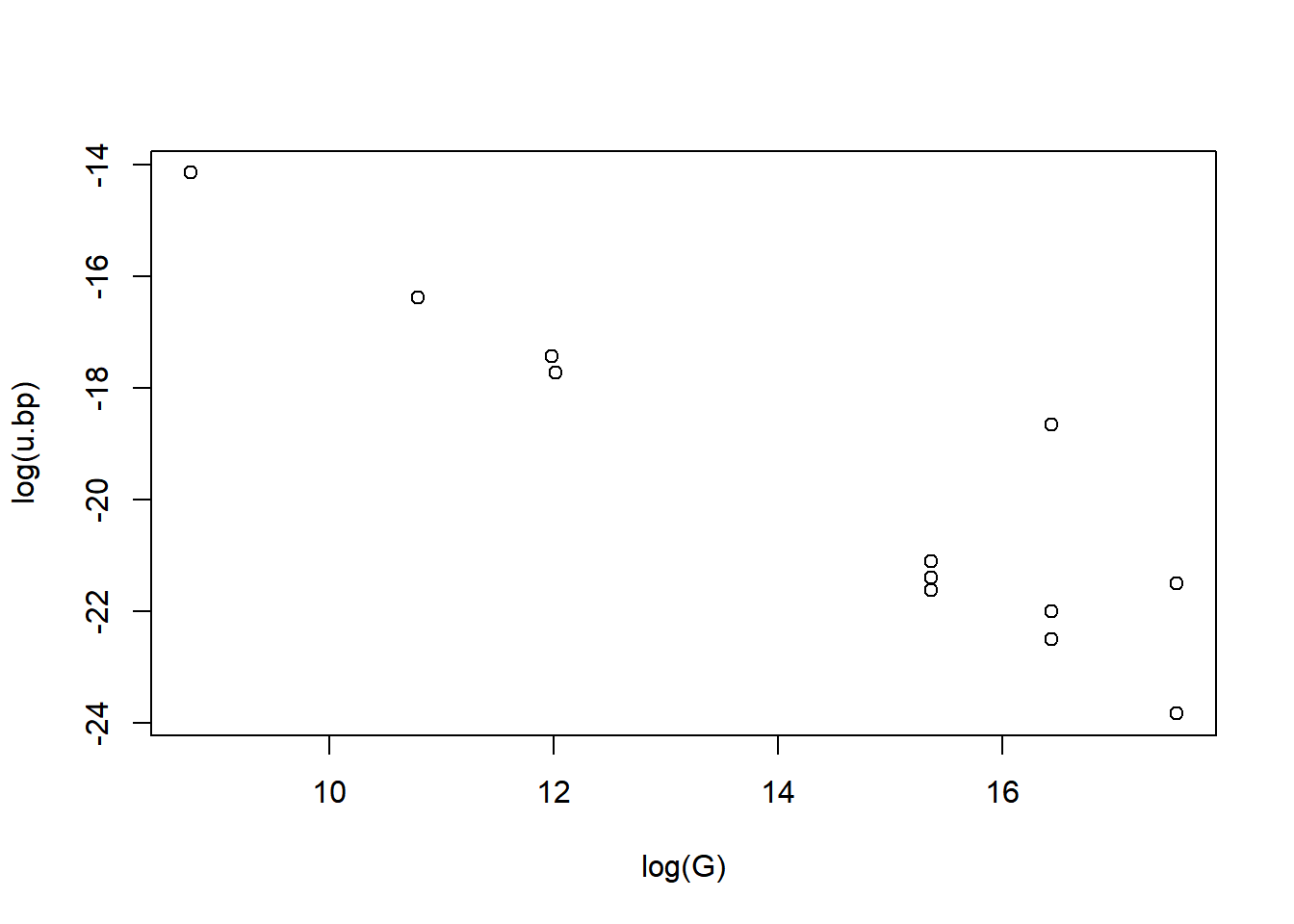Check out the y axis and the x axis of the plot and compare it to the figure (get the figure at https://www.pnas.org/content/88/16/7160 ). What’s wrong?

A: the x and y axis is not the same

R’s default log() functions uses the natural log ln. To do the base 10 log change the code to use log10()

plot(log10(u.bp) ~ log10(G), data = table1)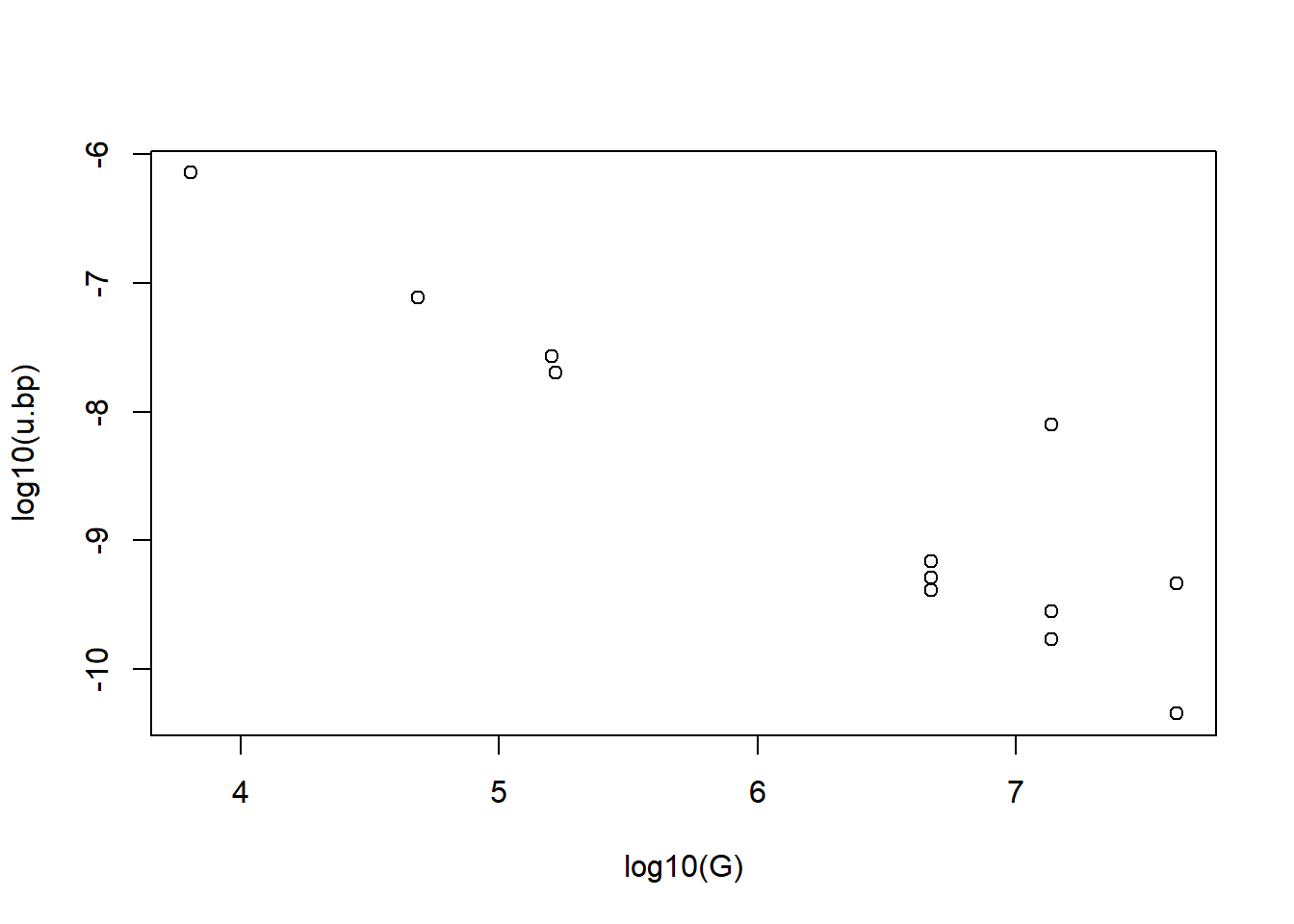## Make Figure 1 with ggpubr

ggpubr makes nicer plots than base R, but you can’t nest functions in it. So what we need to do is make a column of the logged variables. For Genome size it requires this:

table1$log10.G <- log10(table1$G)

Now make a new column for logged u.bp, using log10. Call it log10.u.bp. Try to type it out yourself first.

Here’s the code if you need to copy and paste it:

table1$$log10.u.bp <- log10(table1$$u.bp)

table1$log10.u.bp <-log10(table1$u.bp)

While we’re at it lets make a log10 mutation rate per genome column called log10.u.g

table1$$log10.u.g <- log10(table1$$u.g) 

table1$log10.u.g <- log10(table1$u.g) 

Check our columns

summary(table1\$log10.u.bp)
##    Min. 1st Qu.  Median    Mean 3rd Qu.    Max.
## -10.347  -9.429  -9.227  -8.623  -7.666  -6.143

We can make scatter plot using ggscatter

library(ggpubr)
ggpubr::ggscatter(data = table1,
y = "log10.u.bp",
x = "log10.G")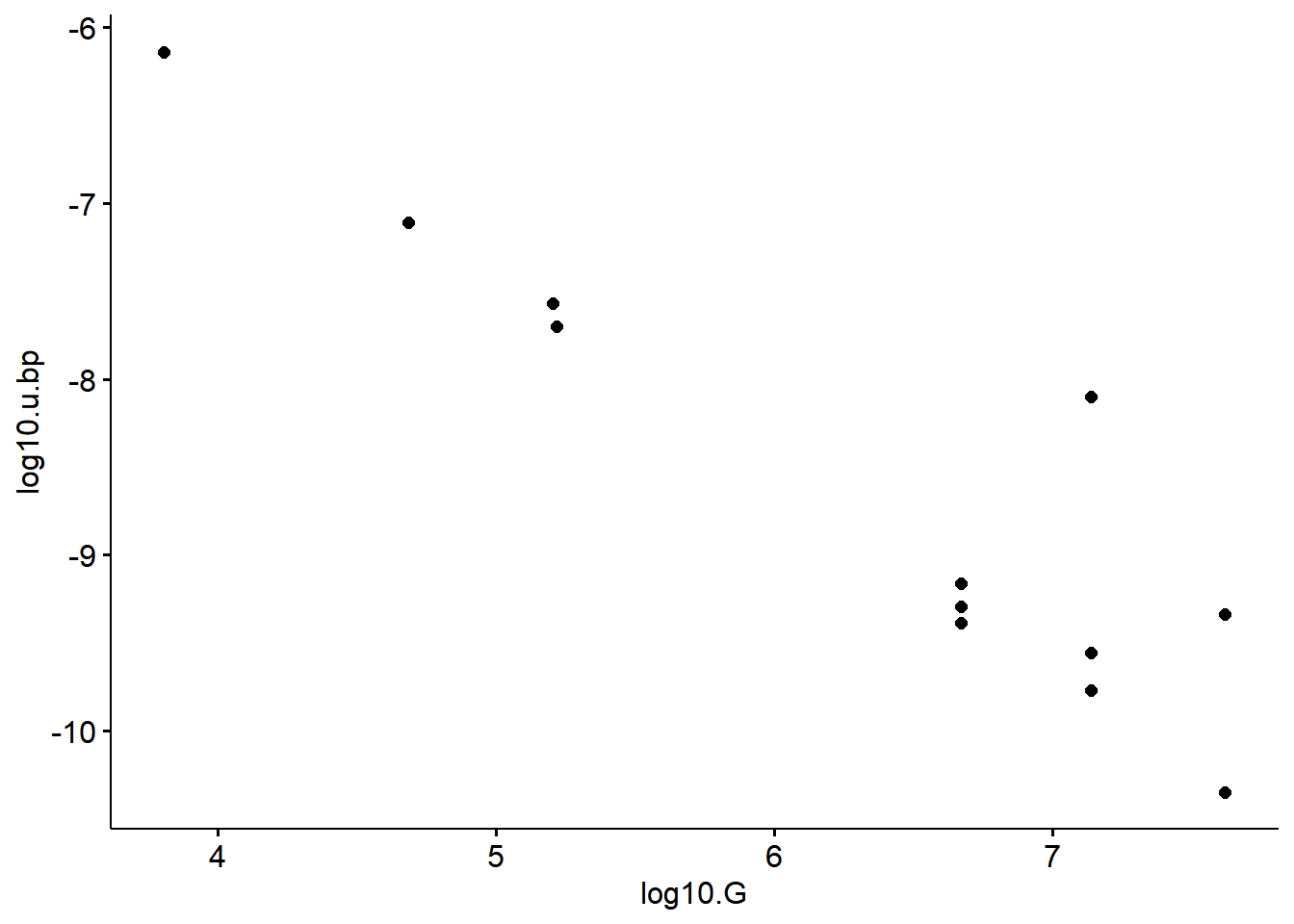We can make lots of nice tweaks with ggpubr. Let’s vary the size of our data points based on the log of the mutation rate per genome, log10.u.g. This lets assess another pattern on top of the main correlation between G and u.bp

ggscatter(data = table1,
y = "log10.u.bp",
x = "log10.G",
size = "log10.u.g") #size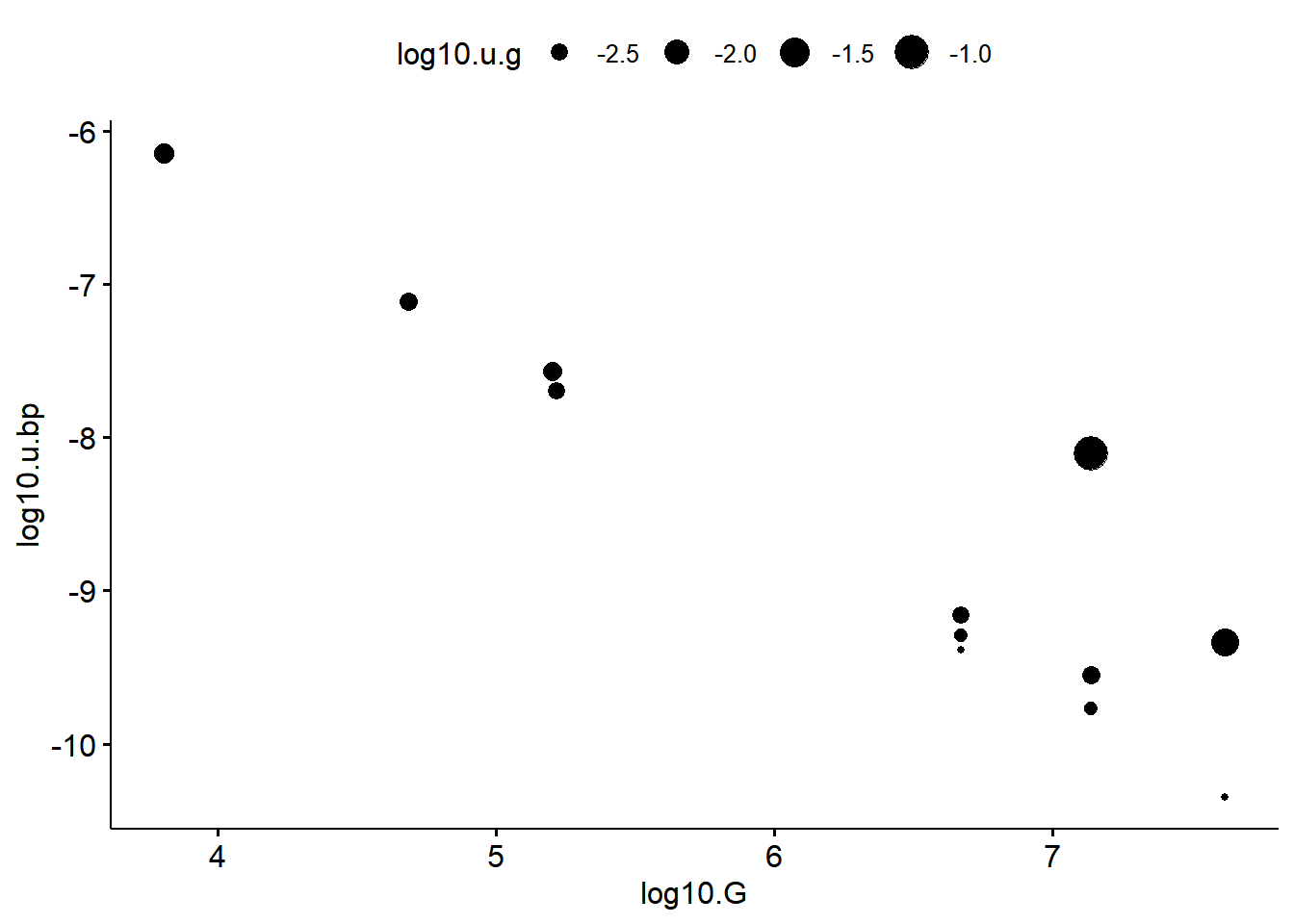Now let’s add color based on log10.u.g also

ggscatter(data = table1,
y = "log10.u.bp",
x = "log10.G",
size = "log10.u.g",   #size
color = "log10.u.g")  #color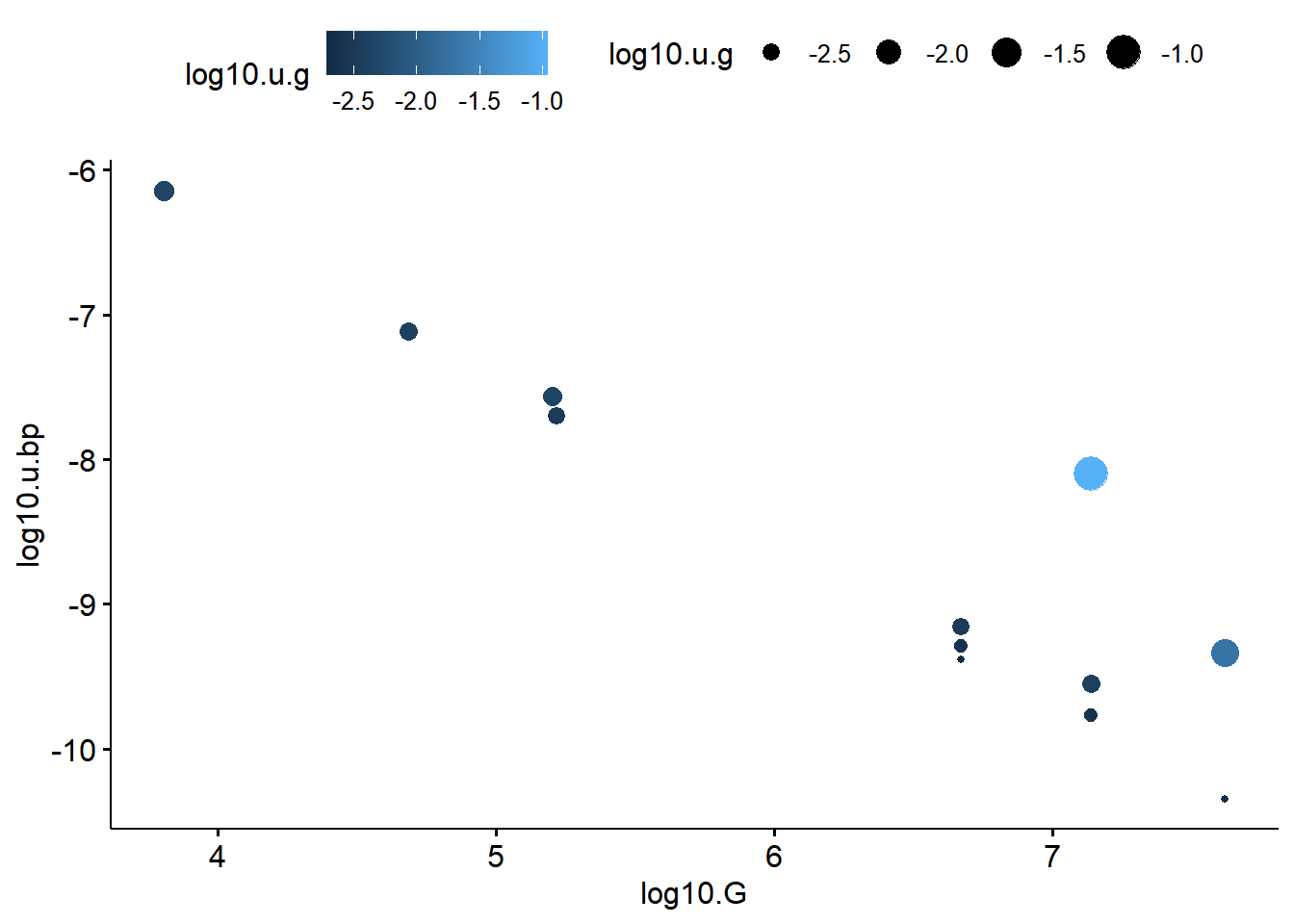The x axis isn’t very clear using the normal column name. Can you spot what is different about the code below?

ggscatter(data = table1,
y = "log10.u.bp",
x = "log10.G",
size = "log10.u.g",
color = "log10.u.g") +
xlab("log(Genome size)")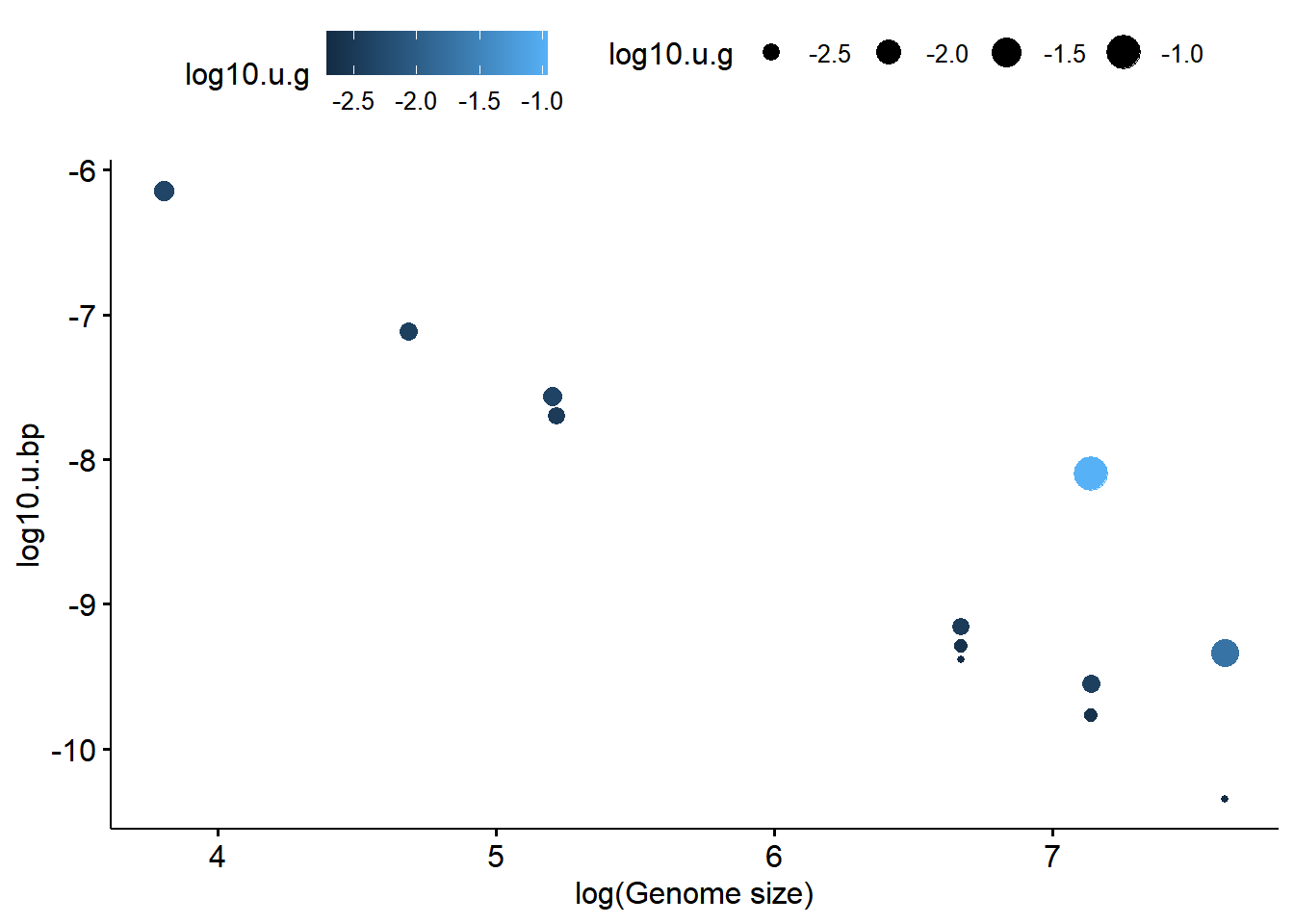Now let’s re-label the y axis. Note the plus signs afer the lines with xlab() and ylab(). What do you think they are doing?

ggscatter(data = table1,
y = "log10.u.bp",
x = "log10.G",
size = "log10.u.g",
color = "log10.u.g") +
xlab("log(Genome size)")     +
ylab("log(mutations per bp)")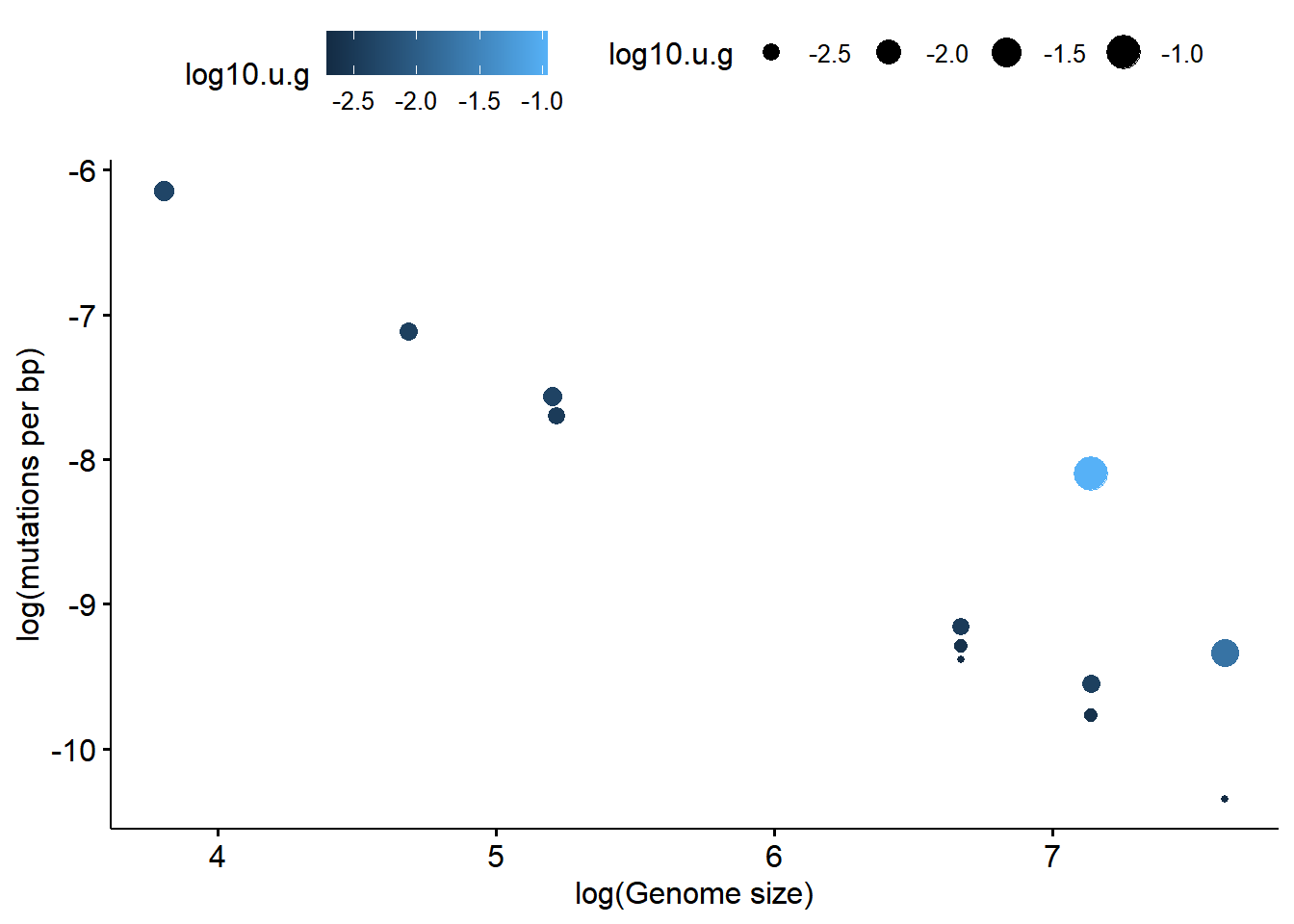There is a strong correlation between genome size and mutation rate (on the log scale). We can further emahsize this patern with a regression line. Note that within the call to ggscatter() I’ve added add = “reg.line”

ggscatter(data = table1,
y = "log10.u.bp",
x = "log10.G",
size = "log10.u.g",
color = "log10.u.g",
xlab("log(Genome size)")     +
ylab("log(mutations per bp)")
## geom_smooth() using formula 'y ~ x'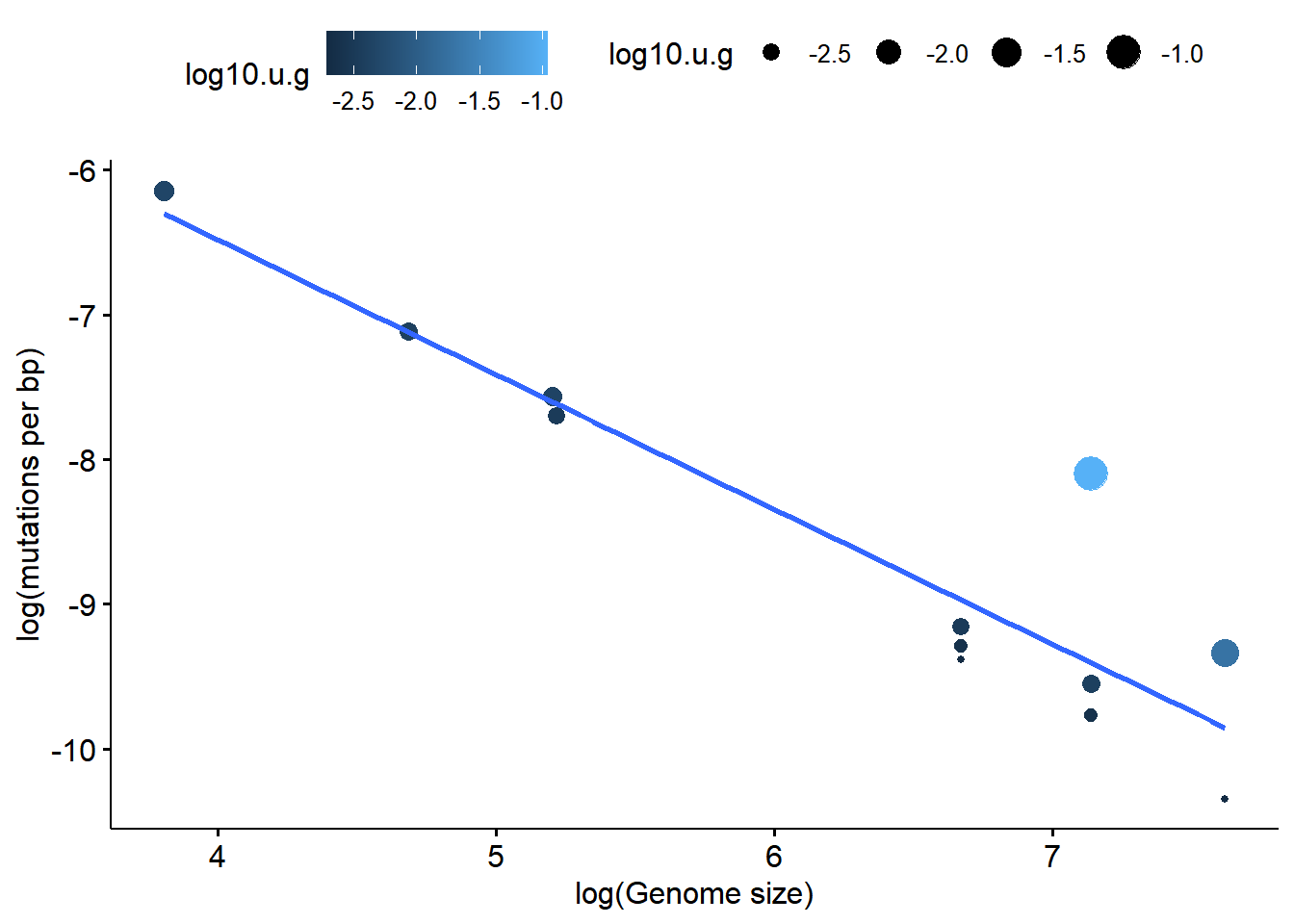We can also fit curved lines easily if we want. See if you can figure out what’s different about this plot. (The line I’ve added is called a smoother made using a specific statistical method called loess.)

ggscatter(data = table1,
y = "u.bp",
x = "log10.G",
size = "log10.u.g",
color = "log10.u.g",
xlab("log(Genome size)")     +
ylab("log(mutations per bp)")
## geom_smooth() using formula 'y ~ x'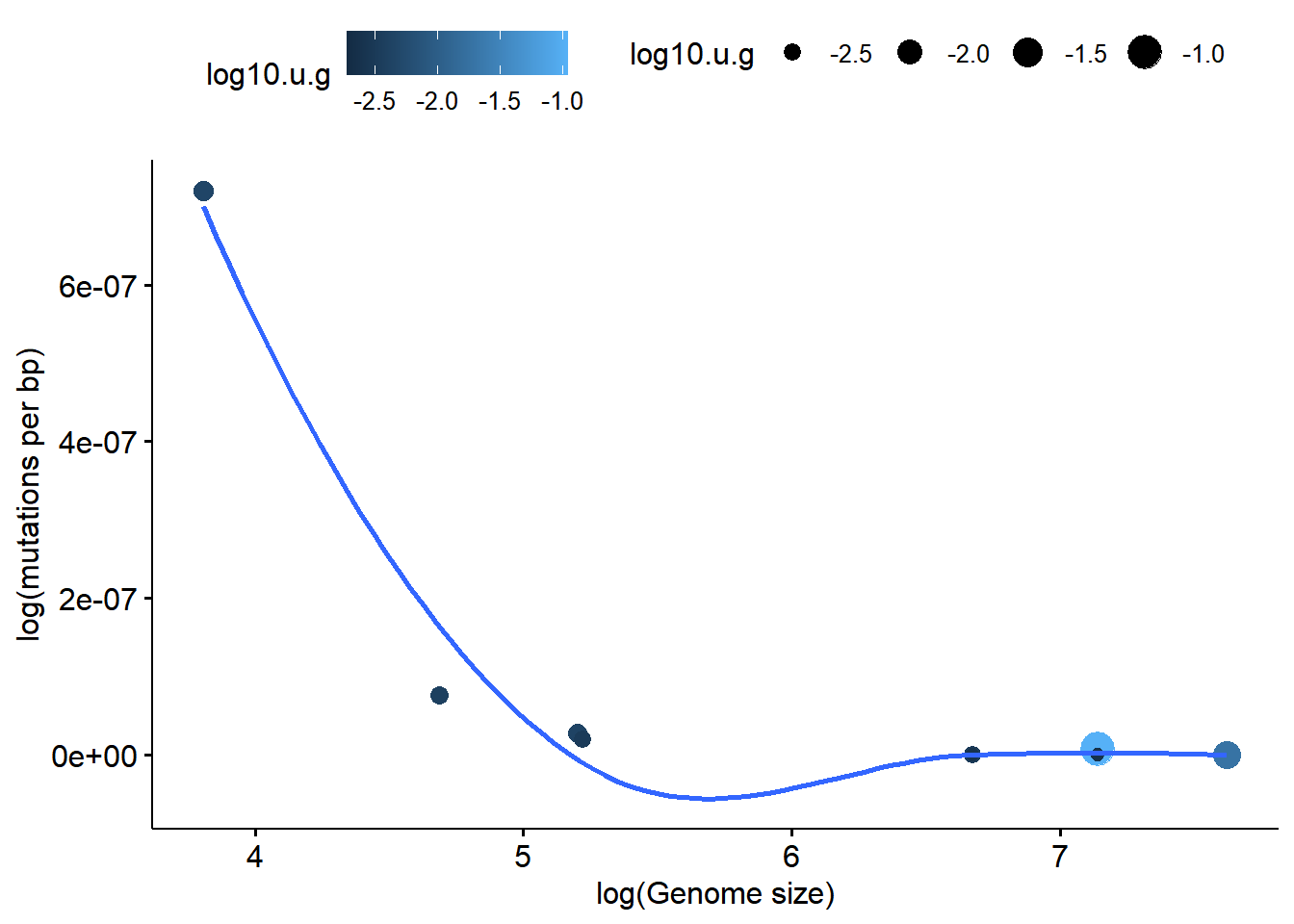We can do some quick statistics on the fly here by adding cor.coef =TRUE. What this does is calcualtes the strength of the relationsip between the x and the y variable (on the log) scale, quantifies it with a statistics R, and calculates stastitically a p-value to give us a sense of whether R is not 0. This is a very strong relationship, so R is almost -1 (R varies between -1 and 1) and the p-value is very small.

(Note that the technical definition of a p-value has a very precise meaning; generally consider p < 0.05 to be “significant”. This means that the pattern we’re seeing in the data is unlikely to have occurred due to chance; however, is does not mean that if we repeated the study with a new data set that we woudln’t get a different result. That is, it doesn’t mean that our conclusions are true!)

ggscatter(data = table1,
y = "log10.u.bp",
x = "log10.G",
size = "log10.u.g",
color = "log10.u.g",
ylab("log(mutations per bp)")
## geom_smooth() using formula 'y ~ x'`